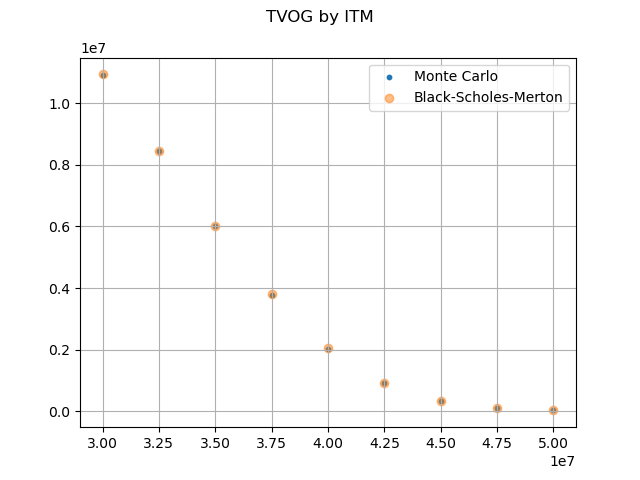# Monte Carlo vs Black-Scholes-Merton¶

Time values of options and guarantees for various in-the-moneyness are calculated using Monte Carlo simulations and the Black-Scholes-Merton pricing formula for European put options.

The Black-Scholes-Merton pricing formula for European put options can be expressed as below, where $$X$$ and $$S_{0}$$ correspond to the sum assured and the initial account value in this example.

\begin{align}\begin{aligned}p=Xe^{-rT}N\left(-d_{2}\right)-S_{0}N\left(-d_{1}\right)\\d_{1}=\frac{\ln\left(\frac{S_{0}}{X}\right)+\left(r+\frac{\sigma^{2}}{2}\right)T}{\sigma\sqrt{T}}\\d_{2}=d_{1}-\sigma\sqrt{T}\end{aligned}\end{align}

The graph below shows the results obtained from the Monte Carlo simulations with 10,000 risk neutral scenarios, and from the Black-Scholes-Merton formula.

Reference: Options, Futures, and Other Derivatives by John C.Hullimport modelx as mx
import pandas as pd
import matplotlib.pyplot as plt
from scipy.stats import norm, lognorm
import numpy as np

proj = model.Projection
proj.model_point_table = proj.model_point_moneyness
monte_carlo = pd.Series(proj.pv_claims_over_av('MATURITY'), index=proj.model_point().index)
monte_carlo = list(np.average(monte_carlo[i]) for i in range(1, 10))